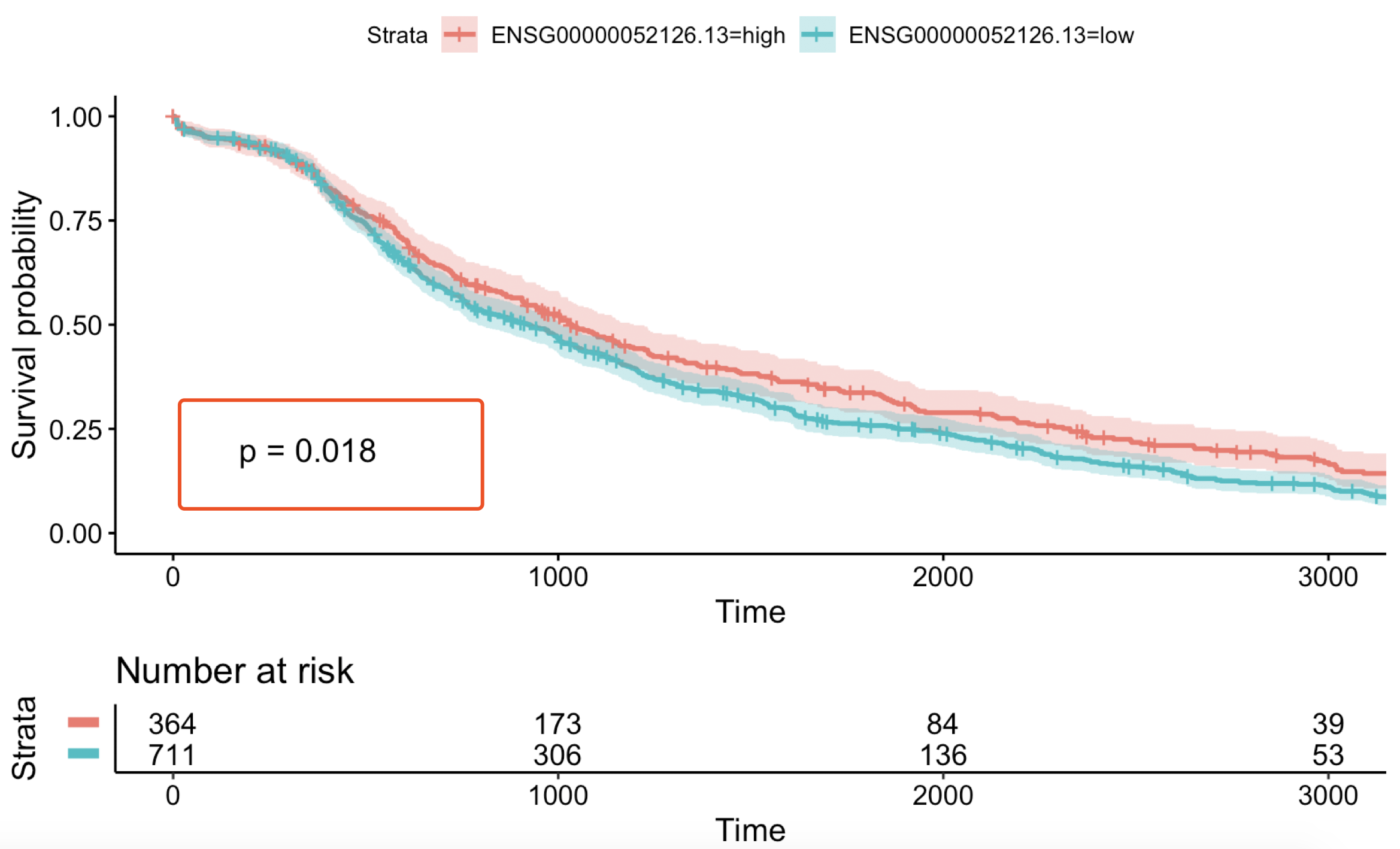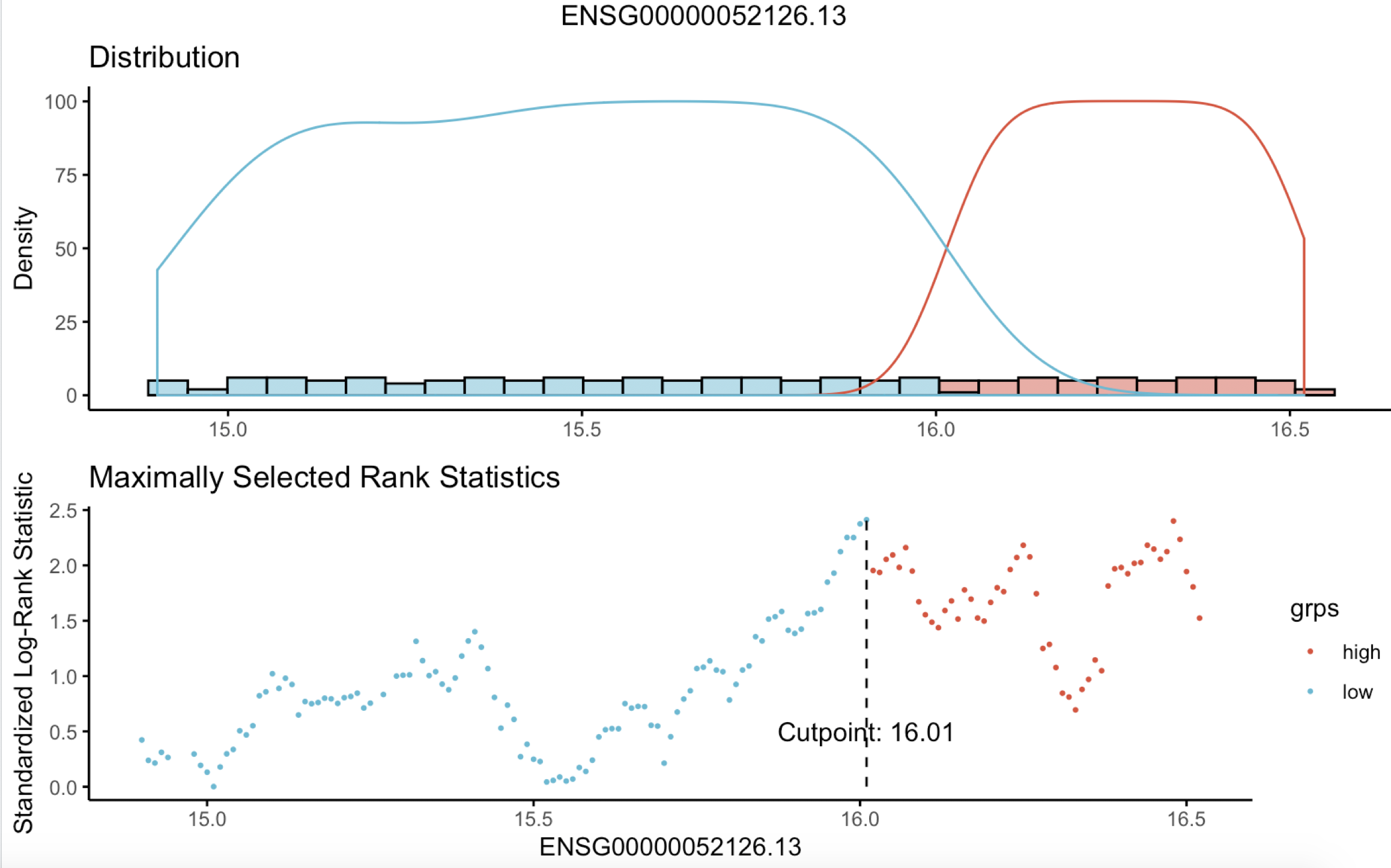# 生存分析就是一个任人打扮的小姑凉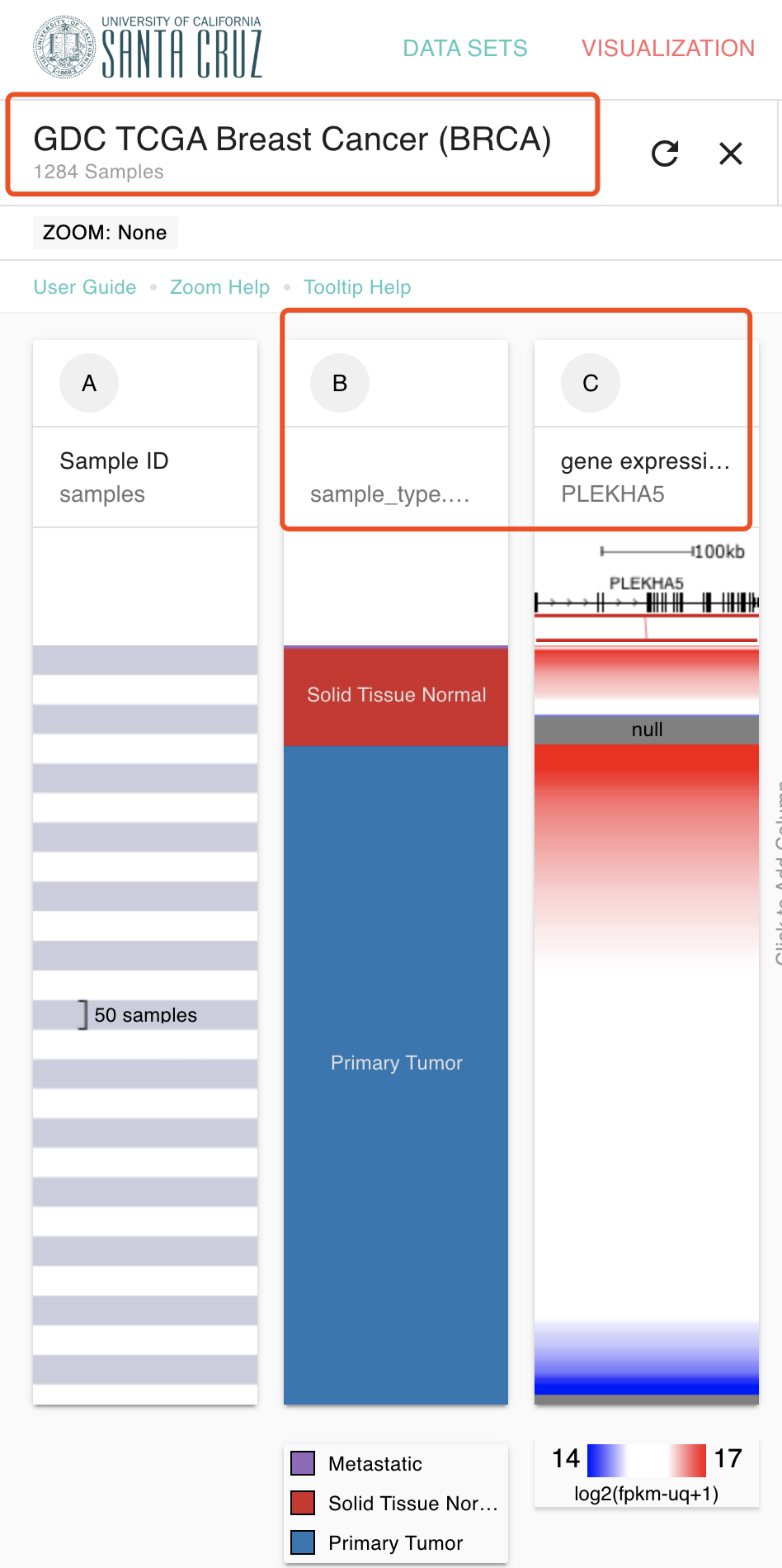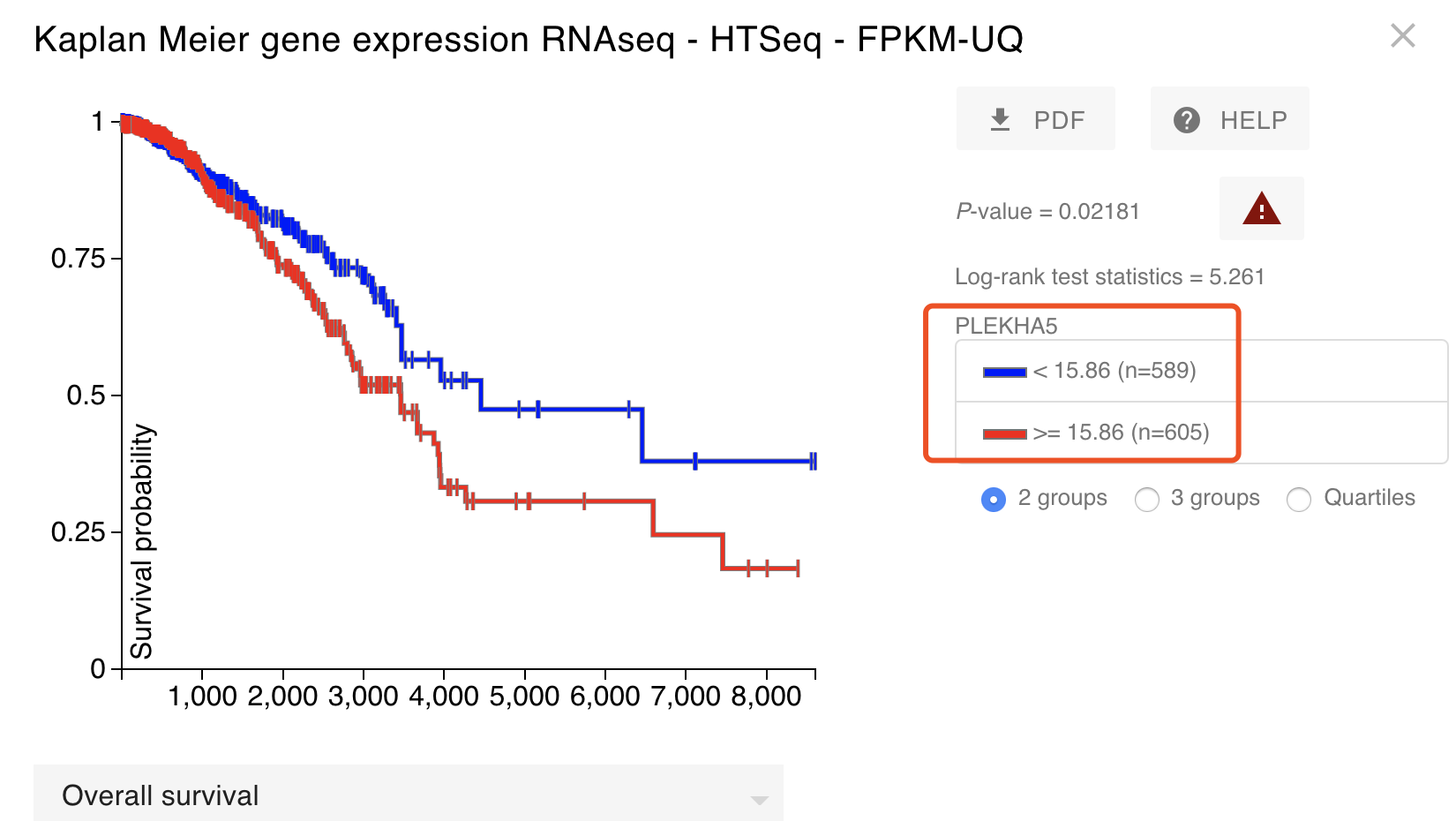(@ο@) 哇～非常显著，差点准备交差了，然后下意识的看了看病人数量，TCGA数据库的BRCA病人没道理居然快1200个了，肯定是有什么地方错误了，重新看了看，的确是因为没有顾虑到里面有正常组织测序的那些病人，怎么说呢，相当于把有正常组织测序的那一百多个病人，在我这个生存分析里面计算了两次，他们的生存时间信息，生存状态都重复计算了，所以实际上这个生存分析是错误的。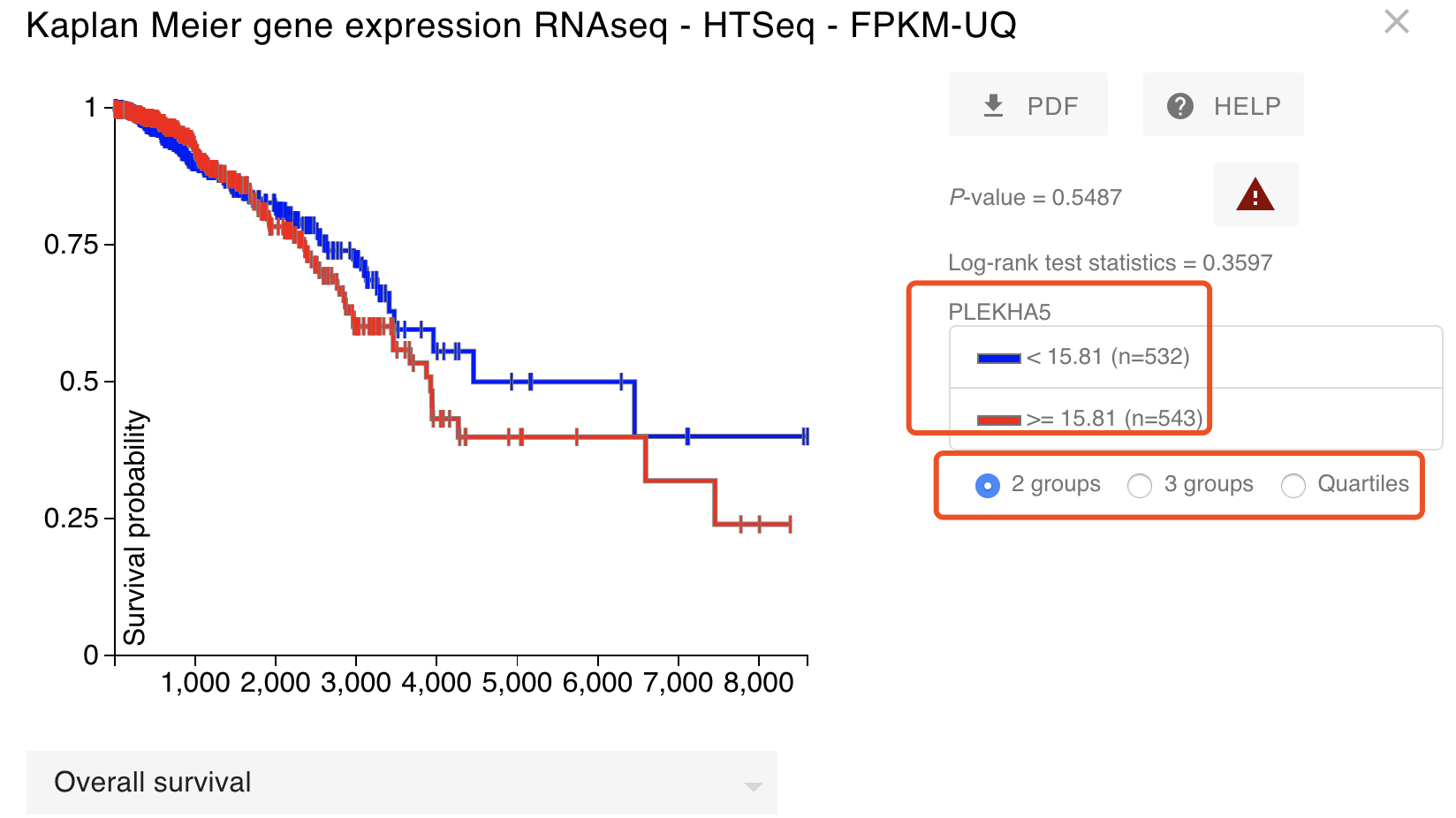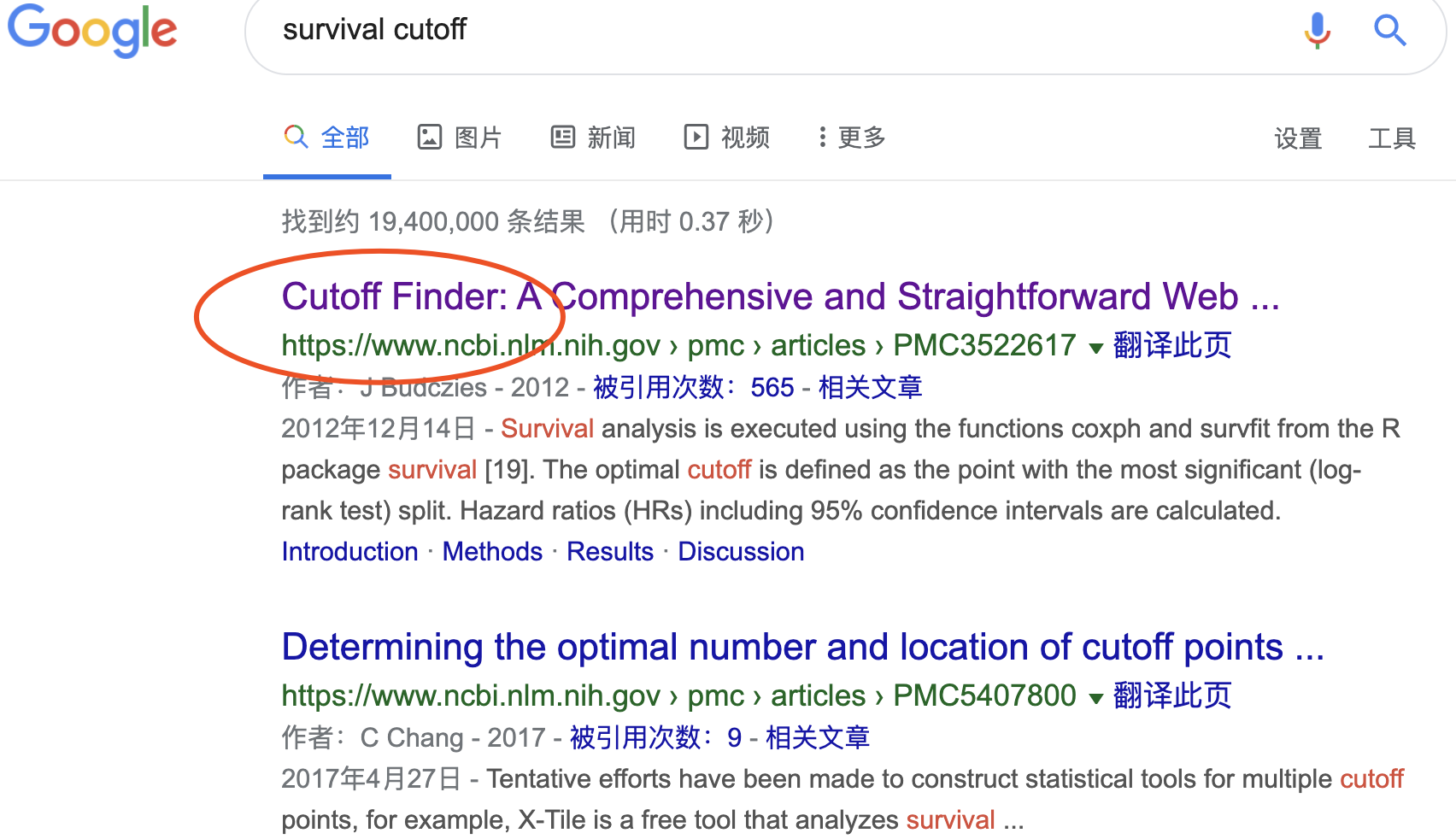### 使用survminer包的surv_cutpoint函数找寻最近生存分析阈值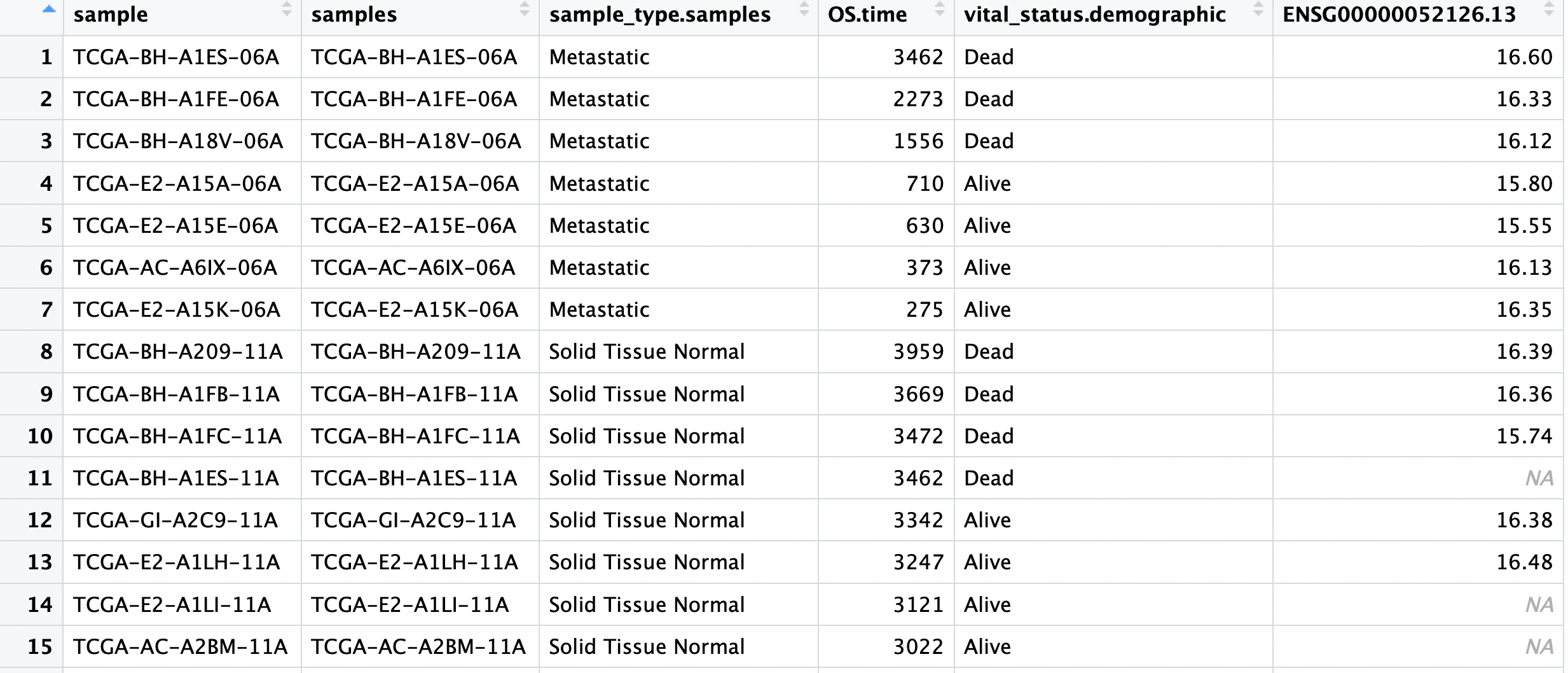``````rm(list=ls())
options(stringsAsFactors = F)
# install.packages("survminer")
library(survminer)
dat=a[a\$sample_type.samples=='Primary Tumor',4:6]
dat\$vital_status.demographic=ifelse(dat\$vital_status.demographic=='Alive',1,0)
surv_rnaseq.cut <- surv_cutpoint(
dat,
time = "OS.time",
event = "vital_status.demographic",
variables = c("ENSG00000052126.13")
)
summary(surv_rnaseq.cut)
plot(surv_rnaseq.cut, "ENSG00000052126.13", palette = "npg")

surv_rnaseq.cat <- surv_categorize(surv_rnaseq.cut)

library(survival)
fit <- survfit(Surv(OS.time, vital_status.demographic) ~ ENSG00000052126.13,
data = surv_rnaseq.cat)
ggsurvplot(
fit, # survfit object with calculated statistics.
risk.table = TRUE, # show risk table.
pval = TRUE, # show p-value of log-rank test.
conf.int = TRUE, # show confidence intervals for
# point estimaes of survival curves.
xlim = c(0,3000), # present narrower X axis, but not affect
# survival estimates.
break.time.by = 1000, # break X axis in time intervals by 500.
risk.table.y.text.col = T, # colour risk table text annotations.
risk.table.y.text = FALSE # show bars instead of names in text annotations
# in legend of risk table
)
``````

`````` time = "OS.time",
event = "vital_status.demographic",
variables = c("ENSG00000052126.13")
``````